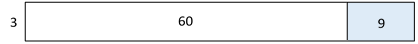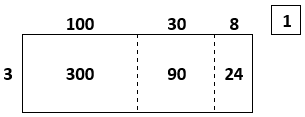# Multiplication and Division of Whole Numbers

## Objective

Divide two-digit, three-digit, and four-digit dividends by one-digit divisors.

## Common Core Standards

### Core Standards

?

• 5.NBT.B.6 — Find whole-number quotients of whole numbers with up to four-digit dividends and two-digit divisors, using strategies based on place value, the properties of operations, and/or the relationship between multiplication and division. Illustrate and explain the calculation by using equations, rectangular arrays, and/or area models.

?

• 4.NBT.B.4

• 4.NBT.B.6

• 5.NBT.A.1

• 5.NBT.A.2

## Criteria for Success

?

1. Compute two-digit by one-digit, three-digit by one-digit, and four-digit by one-digit quotients using the area model, the partial products algorithm, and the standard algorithm.
2. Relate the area model, the partial products algorithm, and the standard algorithm.
3. Estimate two-digit by one-digit, three-digit by one-digit, and four-digit by one-digit quotients.
4. Use the relationship between multiplication and division to check whether one’s answer is correct.

## Tips for Teachers

?

Students have seen the area model and the standard algorithm strategies for all computational types presented in this lesson in Grade 4. The intention of this lesson it to solidify the standard algorithm with these cases before moving onto more cognitively complex cases in later lessons.

#### Fishtank Plus

• Problem Set
• Student Handout Editor
• Vocabulary Package

?

### Problem 1

Find the missing side length of each of the rectangles below. Then find their combined length.#### References

EngageNY Mathematics Grade 4 Mathematics > Module 3 > Topic G > Lesson 33Application Problem

Grade 4 Mathematics > Module 3 > Topic G > Lesson 33 of the New York State Common Core Mathematics Curriculum from EngageNY and Great Minds. © 2015 Great Minds. Licensed by EngageNY of the New York State Education Department under the CC BY-NC-SA 3.0 US license. Accessed Dec. 2, 2016, 5:15 p.m..

Modified by The Match Foundation, Inc.

### Problem 2

Estimate each quotient. Then use any method to solve.

1. $648\div3$
2. $837\div6$

#### References

EngageNY Mathematics Grade 4 Mathematics > Module 3 > Topic G > Lesson 33Concept Development

Grade 4 Mathematics > Module 3 > Topic G > Lesson 33 of the New York State Common Core Mathematics Curriculum from EngageNY and Great Minds. © 2015 Great Minds. Licensed by EngageNY of the New York State Education Department under the CC BY-NC-SA 3.0 US license. Accessed Dec. 2, 2016, 5:15 p.m..

Modified by The Match Foundation, Inc.

### Problem 3

Solve using the standard algorithm. If you get stuck, use an area model and/or the partial products algorithm to help. Then check your answer using multiplication.

1.   $1,344\div6$
2.    $5,216\div4$
3.    $4,309\div7$

#### References

EngageNY Mathematics Grade 4 Mathematics > Module 3 > Topic G > Lesson 33Concept Development

Grade 4 Mathematics > Module 3 > Topic G > Lesson 33 of the New York State Common Core Mathematics Curriculum from EngageNY and Great Minds. © 2015 Great Minds. Licensed by EngageNY of the New York State Education Department under the CC BY-NC-SA 3.0 US license. Accessed Dec. 2, 2016, 5:15 p.m..

Modified by The Match Foundation, Inc.

## Discussion of Problem Set

?

• Look at #1. Which problems were particularly difficult? Why?
• How did you determine what division problem was being solved in #2?
• Look at #4. Are there other division problems with the same quotient and remainder? How do you know?
• Look at #5. What if Mr. Jones has 163 books? How would this change the answer to the question?

?

### Problem 1

Solve. Show or explain your work.

 a.   $72\div6$ b.   $3,809\div5$

### Problem 2

Alyssa is using the area model below to solve a problem.Write the problem represented by the whole area model, including its quotient and remainder.

?